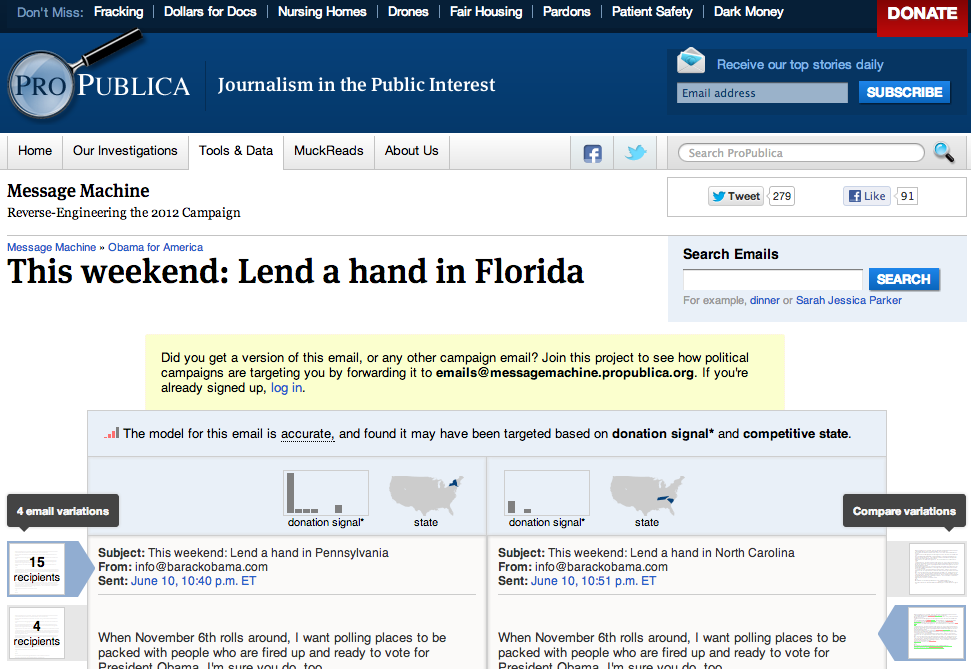# Data Mining Machine Learning

The Message Machine2 Technologies
1. Document Clustering
2. Decision Trees
Decision Trees are Good
because they look like this:
```  dollar_amount >= 101.66 0.4
state >= 33 0.125
dollar_amount >= 227.817 0.5
42252 => {42252=>1.0}
42251 => {42251=>1.0}
42252 => {42252=>10.0}
42251 => {42251=>25.0, 42256=>3.0}
```
and they are non linear.
Decision Tree Basic Algorithm
```build_tree(data)
@best  = Infinity
@left  = []
@right = []
for each @attr in data:
for every @value of @attr
left  = values in @attr < @value
right = values in @attr >= @value
if entropy(left, right) < @best:
@left = left, @right = right
build_tree(@left)
build_tree(@right)
```
Entropy?
You don't have to build your own, there is Weka
Document Clustering and Similarity
The Simple Way: Min Hash
Invented at Altavista
Simple Idea
1. Turn every word in a document into a number via a hash function, and take the lowest number.
2. If 2 documents have the same number, they are similar.
Example:
• "The cat ran" => -1912293768743167748
• "The cat ran away" => -1912293768743167748
• "The dog sat" => -2092219696032009264
Demo
The Best Part?
It can work on any file not just text based documents
The second best part?
One line of code:
```  "The cat ran away".split(/\W+/).map(&:hash).min
```
The Hard More Accurate Way
TF-IDFPairwise comparisons with cosine similaritySimple algorithm, you can get fancier
```cluster(docs)
clusters = [Cluster.new(docs.shift)]
for doc in docs:
for cluster in clusters:
if cosinesim(cluster, doc) > threshold